3 篇文章 1 订阅

# 为什么要做多模态融合的 MOT?

## Motivation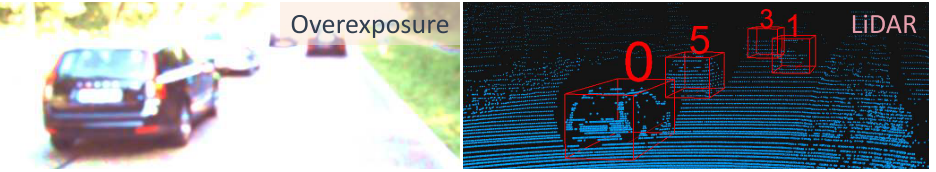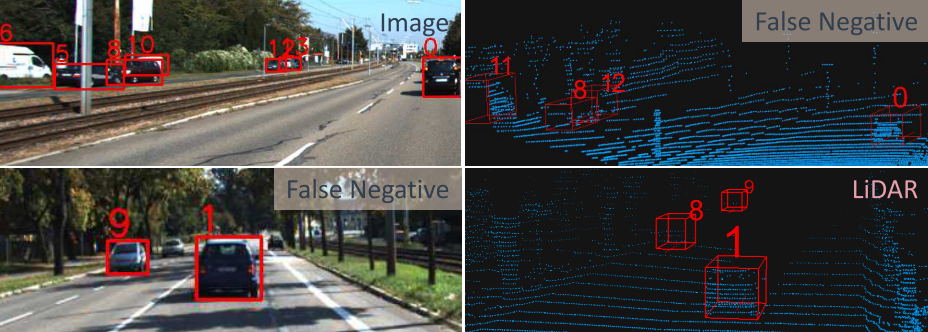## Method

1. object detector:可以选择多个框架例如 PointPillar
2. feature extractor:将各传感器的 feature 提取出来并进行 fusion 送入后端的 adjacency estimator
3. adjacency estimator:这个模块是 modality agnostic 的(可能是因为前面已经融合了 feature 的信息吧)，向后面的 optimizer 输入得分。
4. min-cost flow optimizer:是一个线性规划器(linear programing solver)，根据前面的 各个得分算最优解。

## 问题构造:

X i = { x i i ∣ j = 1 , ⋯   , N } X^{i}=\left\{x_{i}^{i} | j=1, \cdots, N\right\}

X i + 1 = { x k i + 1 ∣ k = 1 , ⋯   , M } X^{i+1}=\left\{x_{k}^{i+1} | k=1, \cdots, M\right\}

1. y j t r u e y_{j}^{t r u e} 指是否为检测是否为 TP

2. y j k link y_{j k}^{\text {link}} 指这个第 j 个 detection 是否和后一帧中第 k 个 detection 属于同一个轨迹中 (trajectory)，相当于是否为同一物体，这些个二进制参数能组成一个第 i 帧的邻接矩阵 A i A_i ，可知 A i A_i ∈ R N × M \in R^{N \times M}

3. y j new , y j end y_{j}^{\text {new}}, y_{j}^{\text {end}} 指第 j 个检测是否为轨迹中新的(最后一个的)检测

Y = [ Y t r u e , Y l i n k , Y n e w , Y e n d ] \mathbf{Y}=\left[Y^{t r u e}, Y^{l i n k}, Y^{n e w}, Y^{e n d}\right]

## 下面是详细介绍:

#### 单模态的 feature extractor: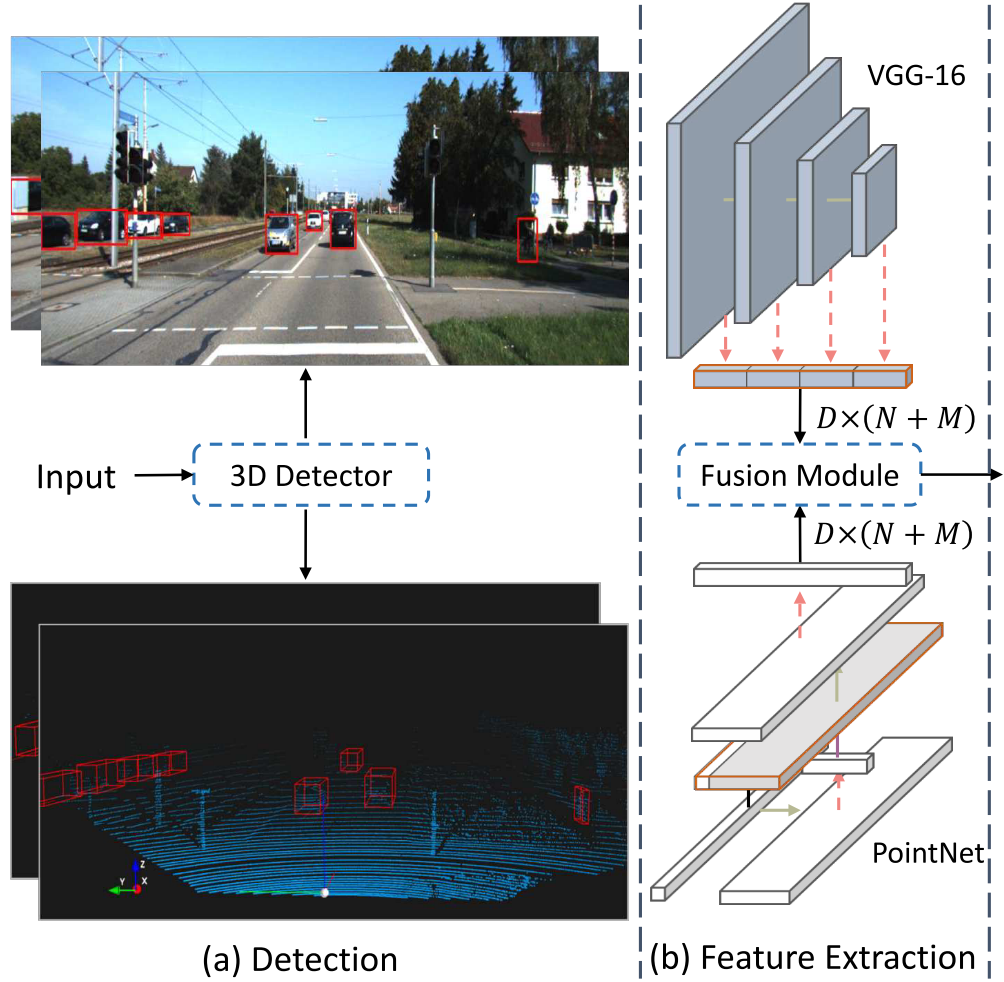#### 多模态 Fusion module: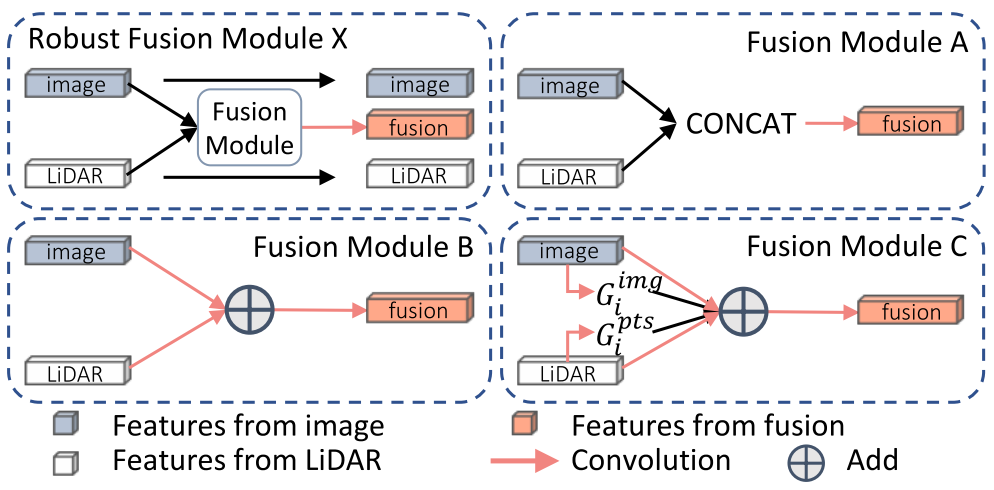A: F i f u s e = W ⊗ CONCAT ⁡ ( F i 0 , ⋯   , F i S ) F_{i}^{f u s e}=W \otimes \operatorname{CONCAT}\left(F_{i}^{0}, \cdots, F_{i}^{S}\right)
B: F i fuse = ( ∑ s = 0 S W s ⊗ F i s ) F_{i}^{\text {fuse}}=\left(\sum_{s=0}^{S} W^{s} \otimes F_{i}^{s}\right)
C: G i s = σ ( W a t t s ⊗ F i s ) G_{i}^{s}=\sigma\left(W_{a t t}^{s} \otimes F_{i}^{s}\right)
F i f u s e = 1 ∑ s = 0 S G i s ∑ s = 0 S G i s ⊙ ( W s ⊗ F i s ) F_{i}^{f u s e}=\frac{1}{\sum_{s=0}^{S} G_{i}^{s}} \sum_{s=0}^{S} G_{i}^{s} \odot\left(W^{s} \otimes F_{i}^{s}\right)

⊗ \otimes 是卷积操作，C 模块有类似 attention 机制参与 ，期望能学到那些 feature 比较重要。

#### 相关性运算:

Element-wise multiplication: F j k = F j i ⊙ F k i + 1 F_{j k}=F_{j}^{i} \odot F_{k}^{i+1}

Subtraction: F j k = F j i − F k i + 1 F_{j k}=F_{j}^{i}-F_{k}^{i+1}

Absolute subtraction: F j k = ∣ F j i − F k i + 1 ∣ F_{j k}=\left|F_{j}^{i}-F_{k}^{i+1}\right|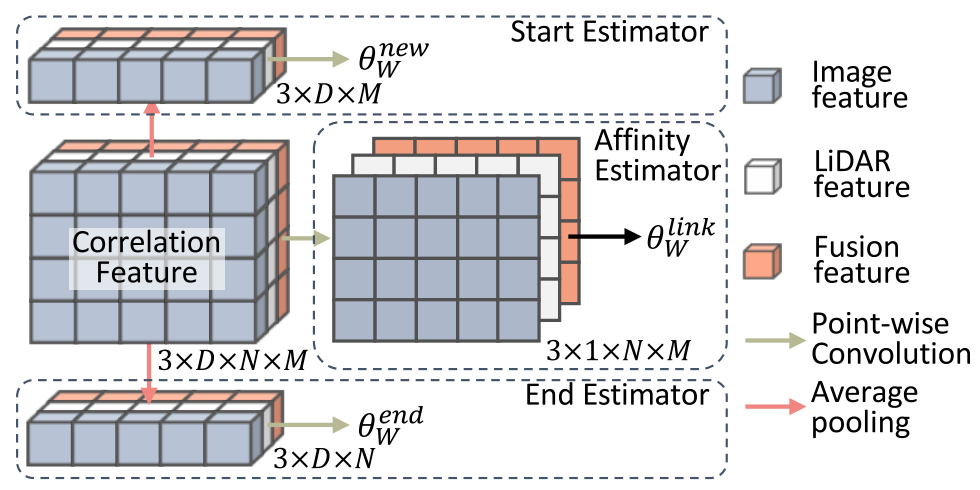Loss function:
L = L l i n k + α L s t a r t + γ L e n d + β L t r u e L=L_{l i n k}+\alpha L_{s t a r t}+\gamma L_{e n d}+\beta L_{t r u e}

#### Linear programming:

(这些都在先前工作有讲，准备看一下后补充)

## 错误分析: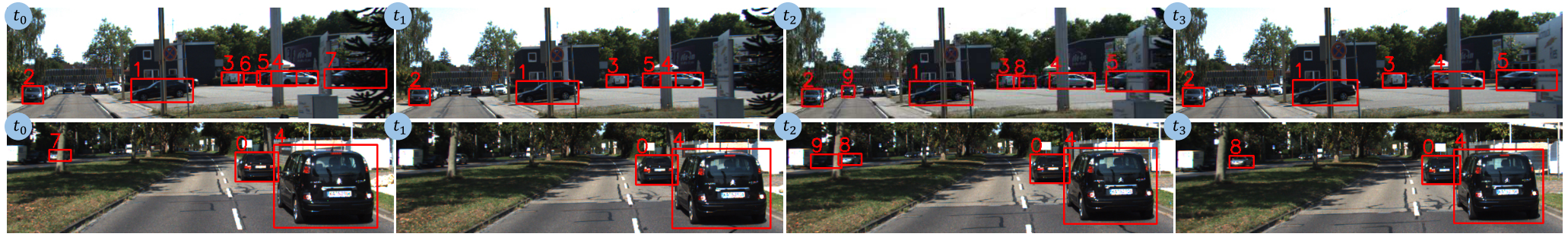2D 检测时经常由于遮挡或光线变化导致误检，就像第一行中的 ID 6，经过一帧 FN 后，突 然就被分配了个 ID 8，或者第二行中的 ID 7 也是这个情况又如第一行中 ID 9 后面没有继续 检测出来。作者说后续应该引入 temporal information 到数据关联中进行预测的强化。 我个人还是感觉，既然有这种情况发生，是因为前端 2D 的 detector 效果不太好并且后续 和 LiDAR 融合的不够好，如果融合够好，且 3D 的 detector 性能好一些是能提出一个比较 robust 的 fusion 特征的，会使得 ID 重新分配情况减少。或者就如同作者所说，加入时序信息，看这个样子感觉是只利用到了前后帧信息.

10-30188301-022875
02-02180
11-29601
11-051182
12-232089
11-07918
11-101万+
08-0282
06-10195
07-12101
07-25621
10-04346
08-2244
04-011839点击重新获取扫码支付余额充值